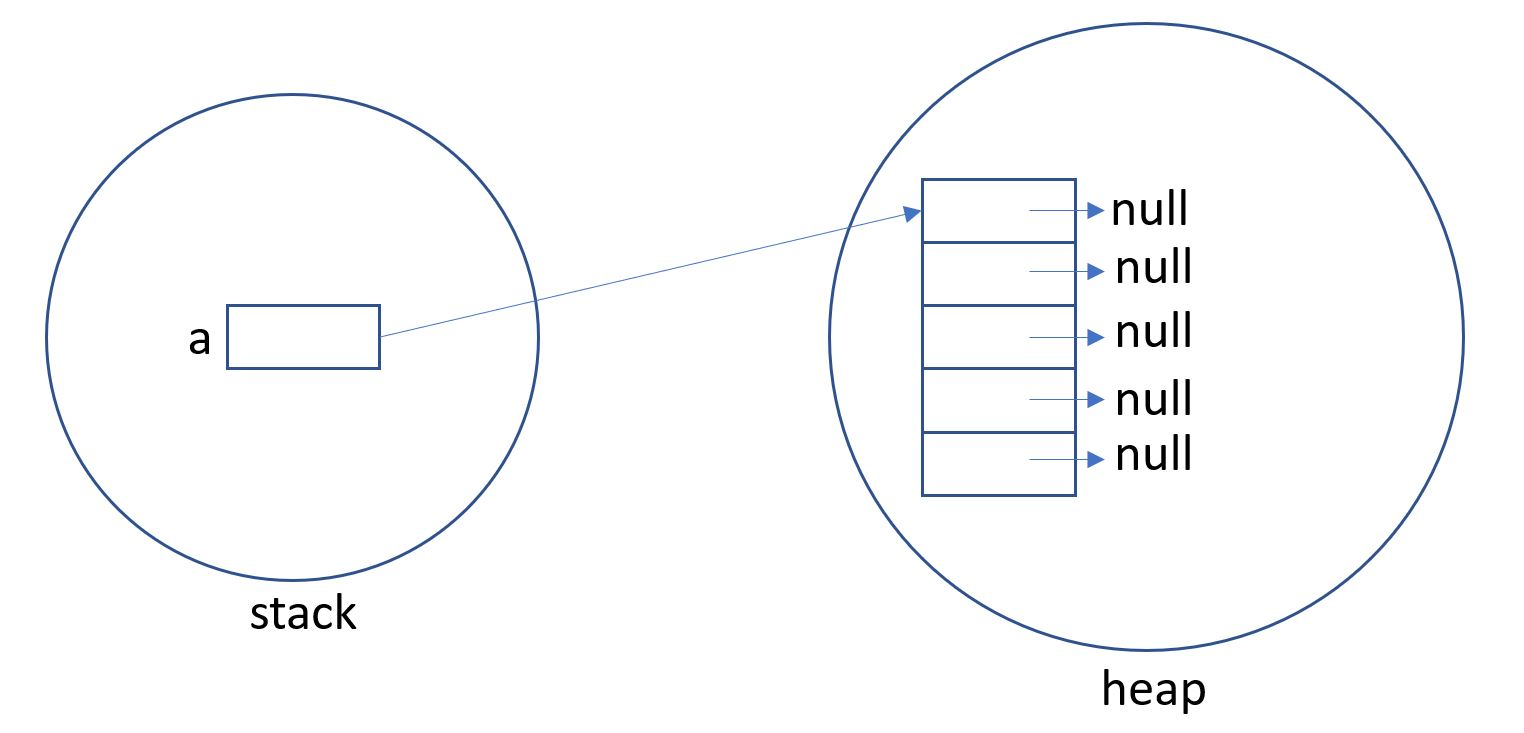# 陣列

在〈陣列〉中留言功能已關閉

Kotlin陣列裏號稱可以存放不同的資料型態。其實是騙人的，因為他是被宣告成 Any

fun main(args:Array<String>) {    var a:Array<Any> = arrayOf("abc", 1, 2, 3)    for (i in a.indices){        println(a[i])    }}

# Array宣告

fun main(args:Array<String>){    var s1:Array<Int> =Array(5, {it -> 0}) //寫於()之內    var s2:Array<Int> =Array(5){it->10} //也可以寫於()之外    s1=100    for (i in s1.indices){        println(s1[i])    }}

# 空元素的陣列宣告

var s1= arrayOfNulls<Int>(5)

var s2= arrayOf<Int>(10,20,30,40,50)

var s3= arrayOf(10,20,30,40,50)

# 宣告基本型態–初始化為0

Kotlin內建一些常用的基本陣列物件，如ByteArray, IntArray, DoubleArray。

var s4=IntArray(5)s4=95s4=100

var s5=intArrayOf(80,90,95,98,96)

# 宣告其他型態

var s6= arrayOfNulls<String>(5)

var s7= arrayOf<String>("thomas", "anna", "John")

var s8= arrayOf("thomas", "anna", "John")

# 陣列存取

## 足一存取

fun main(args:Array<String>){    var s=intArrayOf(5,10,15,20,25)    s=100    s=50    println(s)    println(s)}

## for each

fun main(args:Array<String>){    var ss=intArrayOf(5,10,15,20,25)    for(s in ss){        println(s)    }}

## for (i in arrayVar.indices)

fun main(args:Array<String>){    var ss=intArrayOf(5,10,15,20,25)    for(i in ss.indices){        println(ss[i])    }}

## for (i in 0 until arrayVar.size)

fun main(args:Array<String>){    var ss=intArrayOf(5,10,15,20,25)    for(i in 0 until ss.size){        println(ss[i])    }}

# 二維陣列

fun main(args:Array<String>){    var s3=Array(3, {Array(5, {0})})    s3=10    s3=18    s3=60    for (row in s3){        for (col in row){            print("%2d ".format(col))        }        println()    }}

fun main(args:Array<String>){    var s1=arrayOf(arrayOf(1,2,3), arrayOf(4,5,6,7), arrayOf(9,10,11,12,13))    for (i in s1){        for (j in i){            print("%d ".format(j))        }        println()    }}

# 動態陣列

var a1:Array<Int?> = Array(5,{null})var a2=arrayOfNulls<Int>(5)var a:Array<Int>? = null//待日後決定要產生8個元素，再用如下方式產生陣列a=Array(5, {null})

fun main(args:Array<String>) {    var a:Array<Int?>? = null    //.......    a=Array(5, {null})}

# 全域變數動態陣列

var a:Array<Int?>? = nullfun main(args:Array<String>) {    a=Array(5, {null})    test()會因為這一行讓 a 又變成 null    for (i in a.indices){        a[i]=10    }}fun test(){    a=null}

var a:Array<Int?>? = nullfun main(args:Array<String>) {    a=Array(5, {null})    //test()    for (i in a!!.indices) {        a!!.set(i, 10)    }}fun test(){    a=null}

# .all

.all會將陣列裏的元素傳給 {} 區塊進行 and 判定，只要其中一個不符合資料，就回傳false值。若全部都符合，就傳回ture

fun main(args:Array<String>){    var a=arrayOf<String>("5", "15")    var b=a.all{        it.toInt()>10    }    println(b)}結果:false